# Binary Number System And Conversion Digital Technology Bridging

• Slides: 17Binary Number System And Conversion Digital Technology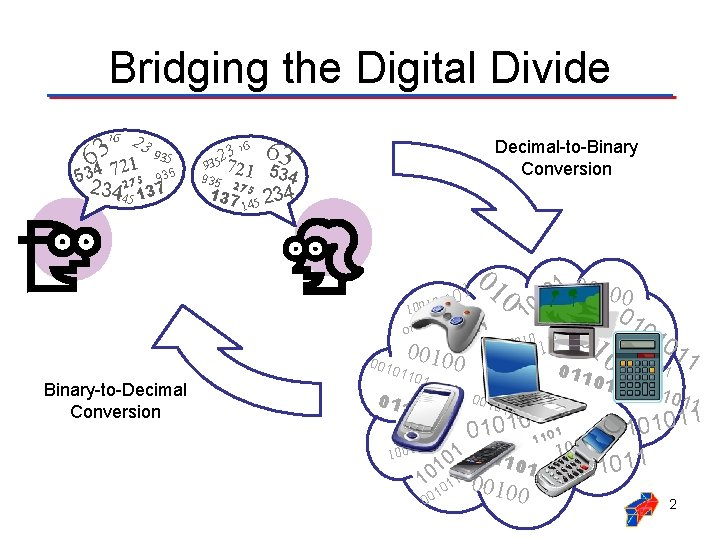Bridging the Digital Divide 16 2 39 3 35 6 1 2 7 534 275 935 234 37 145 1 3 16 63 2 935 72 1 534 935 275 137 145 Decimal-to-Binary Conversion 234 0 1 0010 0 0 1 10 0010110 01 010 01 1 1101 0010 01 0 1 1100 011 11 0 00 001 010 10 0 011 1 01 101 1 011 1101 0 00 0 01 1001 Binary-to-Decimal Conversion 10 0 1 1 011 0 11 100 1 11 0 01 01 010 11 1 1 0 01 1 1 0 100 1 011 1001 0 1 1 011 0 1 1 1 0 0 10 0110001 1 00 00 2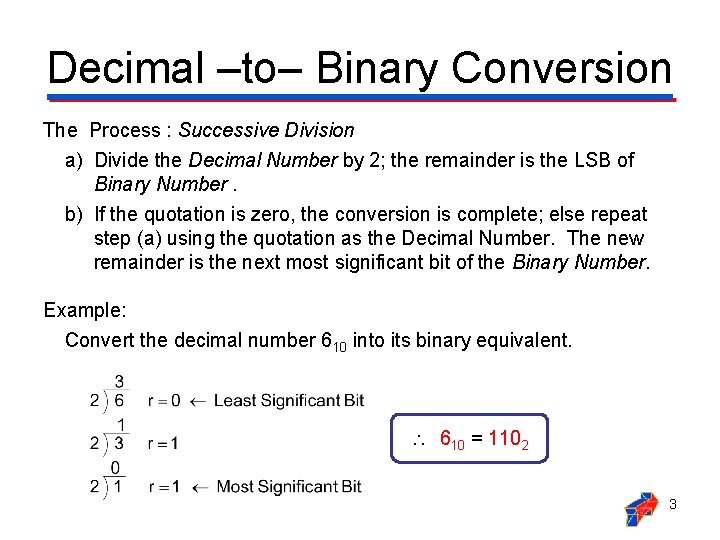Decimal ‒to‒ Binary Conversion The Process : Successive Division a) Divide the Decimal Number by 2; the remainder is the LSB of Binary Number. b) If the quotation is zero, the conversion is complete; else repeat step (a) using the quotation as the Decimal Number. The new remainder is the next most significant bit of the Binary Number. Example: Convert the decimal number 610 into its binary equivalent. 610 = 1102 3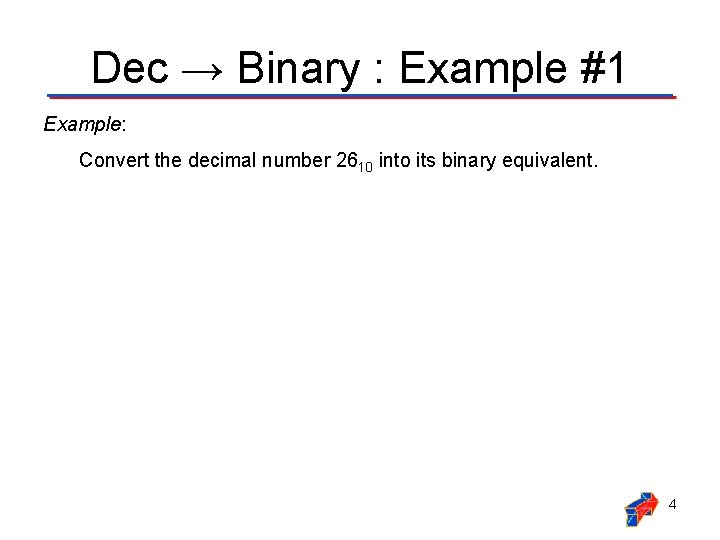Dec → Binary : Example #1 Example: Convert the decimal number 2610 into its binary equivalent. 4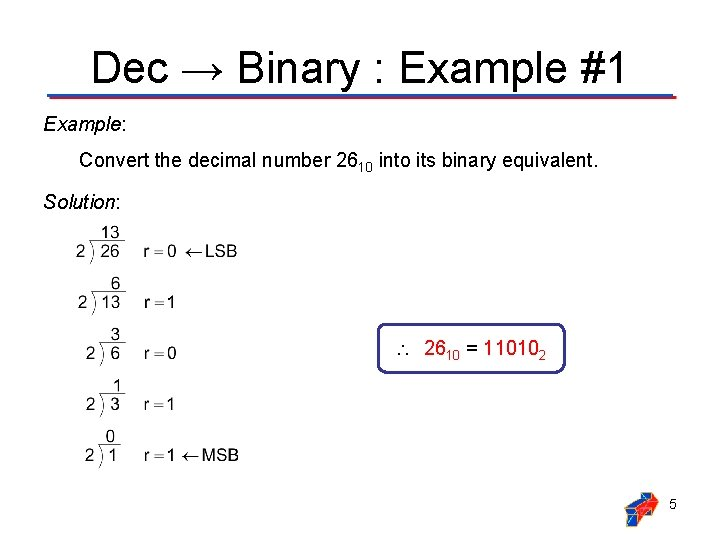Dec → Binary : Example #1 Example: Convert the decimal number 2610 into its binary equivalent. Solution: 2610 = 110102 5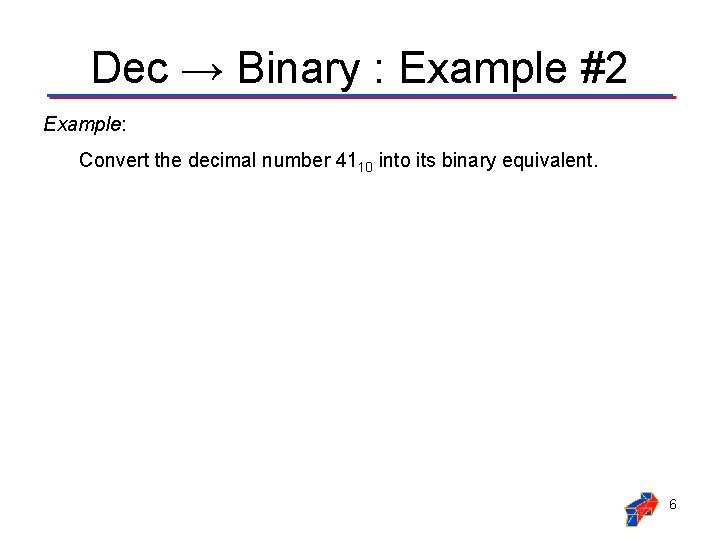Dec → Binary : Example #2 Example: Convert the decimal number 4110 into its binary equivalent. 6Dec → Binary : Example #2 Example: Convert the decimal number 4110 into its binary equivalent. Solution: 4110 = 1010012 7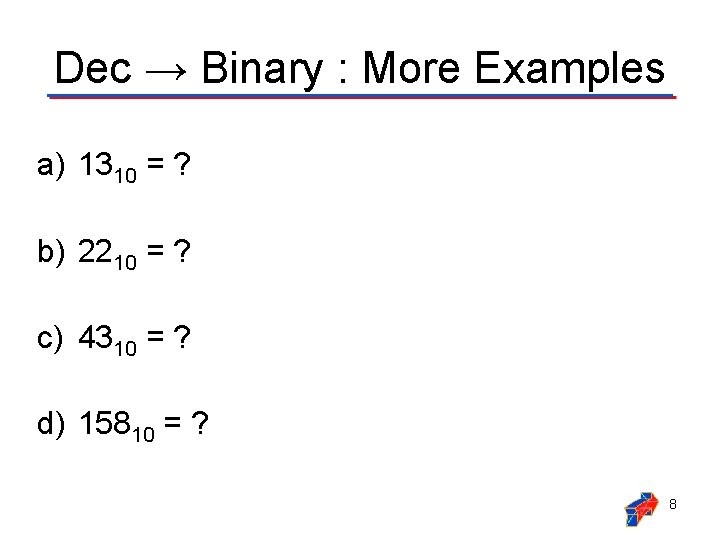Dec → Binary : More Examples a) 1310 = ? b) 2210 = ? c) 4310 = ? d) 15810 = ? 8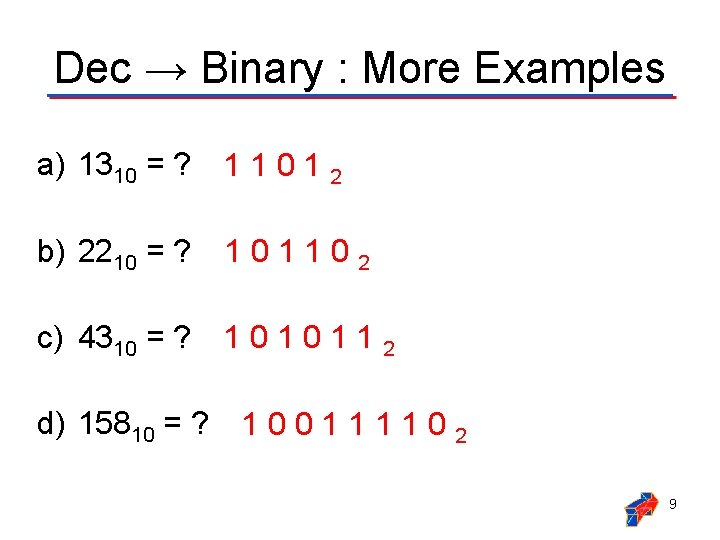Dec → Binary : More Examples a) 1310 = ? 1 1 0 1 2 b) 2210 = ? 101102 c) 4310 = ? 1 0 1 1 2 d) 15810 = ? 100111102 9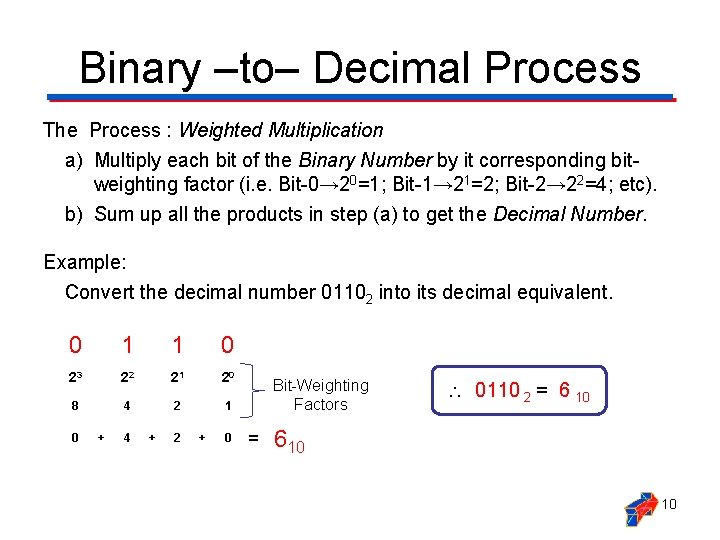Binary ‒to‒ Decimal Process The Process : Weighted Multiplication a) Multiply each bit of the Binary Number by it corresponding bitweighting factor (i. e. Bit-0→ 20=1; Bit-1→ 21=2; Bit-2→ 22=4; etc). b) Sum up all the products in step (a) to get the Decimal Number. Example: Convert the decimal number 01102 into its decimal equivalent. 0 1 1 0 23 22 21 20 8 4 2 1 0 + 4 + 2 + 0 Bit-Weighting Factors = 0110 2 = 6 10 610 10Binary → Dec : Example #1 Example: Convert the binary number 100102 into its decimal equivalent. 11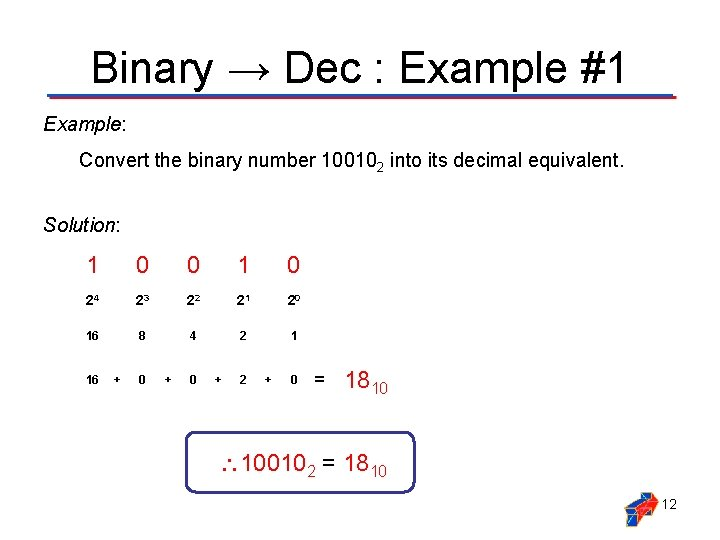Binary → Dec : Example #1 Example: Convert the binary number 100102 into its decimal equivalent. Solution: 1 0 0 1 0 24 23 22 21 20 16 8 4 2 1 16 + 0 + 2 + 0 = 1810 100102 = 1810 12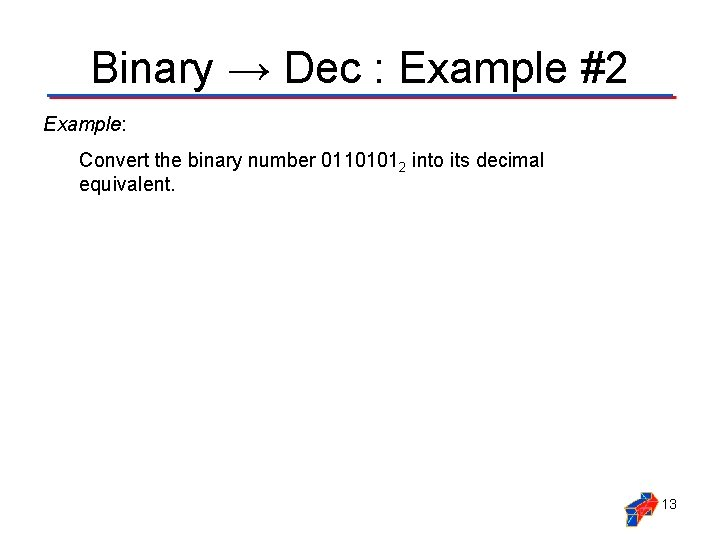Binary → Dec : Example #2 Example: Convert the binary number 01101012 into its decimal equivalent. 13Binary → Dec : Example #2 Example: Convert the binary number 01101012 into its decimal equivalent. Solution: 0 1 1 0 1 26 25 24 23 22 21 20 64 32 16 8 4 2 1 0 + 32 + 16 + 0 + 4 + 0 + 1 = 5310 01101012 = 5310 14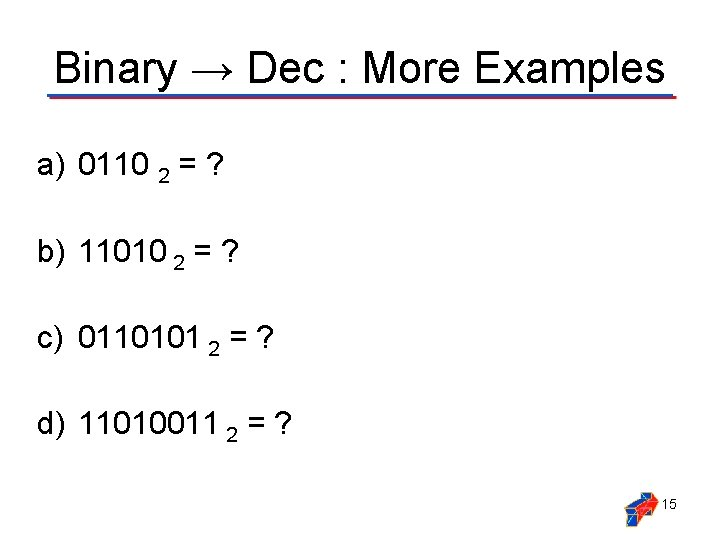Binary → Dec : More Examples a) 0110 2 = ? b) 11010 2 = ? c) 0110101 2 = ? d) 11010011 2 = ? 15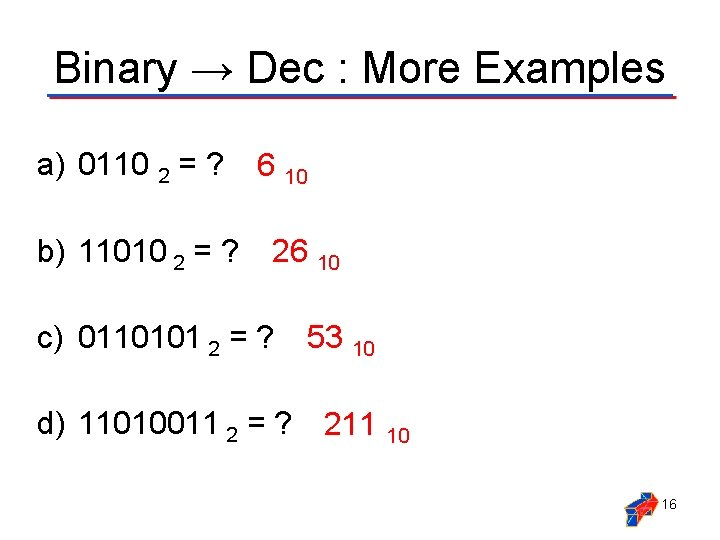Binary → Dec : More Examples a) 0110 2 = ? b) 11010 2 = ? 6 10 26 10 c) 0110101 2 = ? 53 10 d) 11010011 2 = ? 211 10 16Summary & Review Base 10 DECIMAL Successive Division Base 2 BINARY a) Divide the Decimal Number by 2; the remainder is the LSB of Binary Number. b) If the Quotient Zero, the conversion is complete; else repeat step (a) using the Quotient as the Decimal Number. The new remainder is the next most significant bit of the Binary Number. Base 2 BINARY Weighted Multiplication Base 10 DECIMAL a) Multiply each bit of the Binary Number by it corresponding bit-weighting factor (i. e. Bit-0→ 20=1; Bit-1→ 21=2; Bit-2→ 22=4; etc). b) Sum up all the products in step (a) to get the Decimal Number. 17